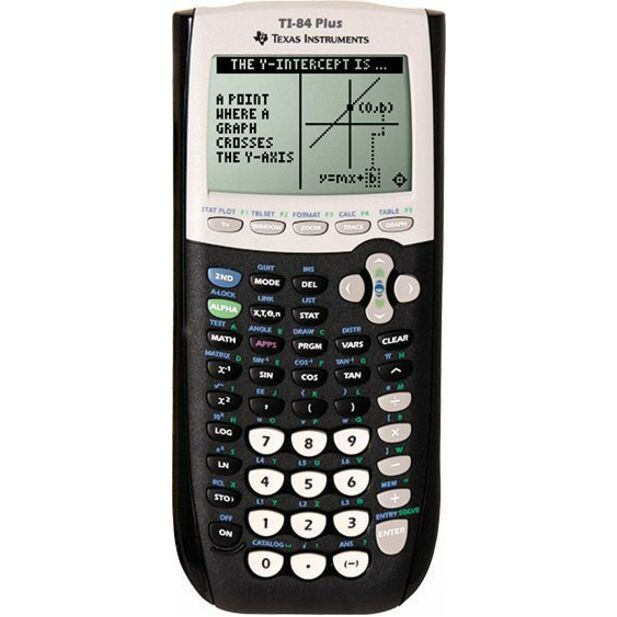Navigate: Home / Hardware / Calculators / Graphing Calculators / Texas Instruments TI-84 Plus Graphics Calculator (On Sale!)

Texas Instruments TI-84 Plus Graphics Calculator (On Sale!)

The TI-84 Plus graphing calculator offers three times the memory, more than twice the speed and a higher contrast screen than the TI-83 Plus model.
*Price: \$149.95 \$109.95
 SKU: TI84PLUS-X Mfr Part #: Other: 84PL/TBL/1L1/A Requires Academic ID: No
Limit one sharing discount per purchase.
 The TI-84 Plus graphing calculator offers three times the memory, more than twice the speed and a higher contrast screen than the TI-83 Plus model. It can be used on the PSAT, SAT, and ACT college entrance exams and AP tests.Expanded graphing technology performance comes preloaded with more than a dozen applications, including functionality that encourages exploration of interactive geometry, inequality graphing and real-world data collection and analysis.The TI-84 Plus graphing calculator is ideal for high school math and science. Its MathPrint™ feature engages students by enabling them to enter fractions and equations in proper notation so they see it on the display exactly as it’s printed in text and on the board. Features include: Take it to The Test(s)Standardized testing organizations have approved the TI-84 Plus for use on the PSAT®, SAT®, and ACT® college entrance exams, IB® Diploma Programme and AP® tests. Carry it from High School to CollegeTexas Instrument (TI) graphing calculators are durable, multi-functional educational tools that meet students’ critical math and science requirements from high school through college. Built-In Functionality Advanced functions accessed through pull-down display menus Real and complex numbers calculated to 14-digit accuracy and displayed with 10 digits plus a two-digit exponent Graphs 10 rectangular functions, six parametric expressions, six polar expressions and three recursively-defined sequences Up to 10 graphing functions defined, saved, graphed and analyzed at one time Sequence graphing mode shows time series plot, cobweb/stair-step plot and phase plots User-defined list names; lists store up to 999 elements Fourteen interactive zoom features Function evaluation table shows numeric evaluation of functions in table format Interactive analysis of function values, roots, maximums, minimums, integrals and derivatives Seven different graph styles for differentiating the look of each graph drawn Horizontal and vertical split-screen options Matrix operations including inverse, determinant, transpose, augment, reduced row echelon form and elementary row operations; convert matrices to lists and vice-versa List-based one- and two-variable statistical analysis, including logistic, sinusoidal, median-median, linear, logarithmic, exponential, power, quadratic polynomial, cubic polynomial, and quadratic polynomial regression models Three statistical plot definitions for scatter plots, xy-line plots, histograms, regular and modified box-and-whisker plots, and normal probability plots Advanced statistics features including 10 hypothesis testing functions, seven confidence interval functions and one-way analysis of variance Fifteen probability distribution functions including Normal, Student-t, Chi-square, Binomial and Poisson Business functions including Time-Value-of-Money (TVM), cash flows, and amortization; full screen interactive editor for solving TVM problems Interactive equation solver editor for solving for different variables in an equation. Alphabetical CATALOG of all TI calculator operations in one menu